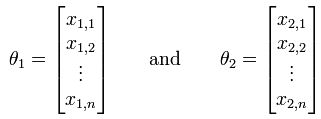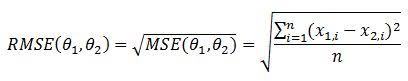### Introduction

The Root Mean Square Error (RMSE) or Root Mean Square Deviation (RMSD) is a frequently used measure of the differences between values predicted by a model or an estimator and the values actually observed. RMSE is a good measure of accuracy. These individual differences are also called residuals, and the RMSE serves to aggregate them into a single measure of predictive power.

#### Formula

Supposed we have the values predicted by an estimator and the values actually observed as follows:Then, the formula is:### References & Resources

• Wikipedia - http://en.wikipedia.org/wiki/Root-mean-square_deviation